# Some Applications of Trigonometry Class 10 Extra Questions Maths Chapter 9 with Solutions Answers

Here we are providing Some Applications of Trigonometry Class 10 Extra Questions Maths Chapter 9 with Answers Solutions, Extra Questions for Class 10 Maths was designed by subject expert teachers.

## Extra Questions for Class 10 Maths Some Applications of Trigonometry with Answers Solutions

Extra Questions for Class 10 Maths Chapter 9 Some Applications of Trigonometry with Solutions Answers

### Some Applications of Trigonometry Class 10 Extra Questions Short Answer Type 1

Question 1.
If a man standing on a platform, 3 metres above the surface of a lake observes a cloud and its reflection in the lake, then the angle of elevation of the cloud is equal to the angle of depression of its reflection.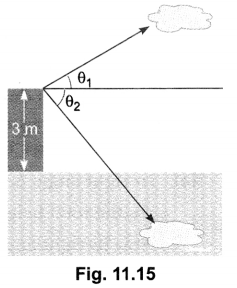Solution:
False, θ1 ≠ θ1 (Fig. 11.15)

Question 2.
Find the angle of elevation of the sun when the shadow of a pole h m high is √3 h m long.
Solution: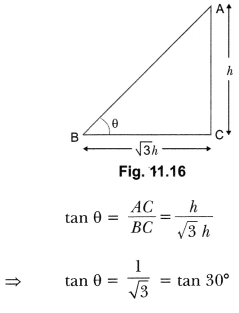In ∆ABC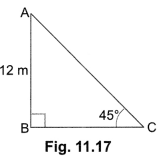θ = 30°

Question 3.
The height of a tower is 12 m. What is the length of its shadow when 10 Sun’s altitude is 45°?
Solution: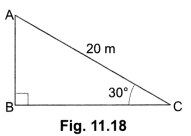Let AB be the tower [Fig. 11.17].
Then, ∠C = 45°, AB = 12 m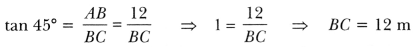∴ The length of the shadow is 12 m.

Question 4.
A circus artist is climbing a 20 m long rope, which is tightly stretched and tied from the top of a vertical pole to the ground. Find the height of the pole, if the angle made by the rope with the ground level is 30° [Fig. 11.18].
Solution:
Let AB be the vertical pole and AC be the long rope tied to point C.
In right ∆ABC, we have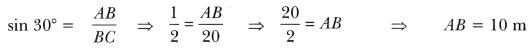Therefore, height of the pole is 10 m.

### Some Applications of Trigonometry Class 10 Extra Questions Short Answer Type 2

Question 1.
The angle of elevation of the top of a tower from a point on the ground, which is 30 m away from the foot of the tower, is 30°. Find the height of the tower.
Solution:
Let BC be the tower whose height is h metres and A be the point at a distance of 30 m from the
foot of the tower. The angle of elevation of the top of the tower from point A is given to be 30°.
Now, in right angle ∆CBA we have,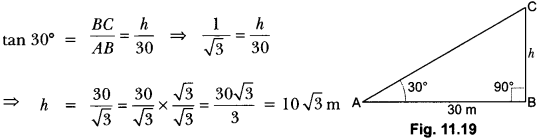Hence, the height of the tower is 10 √3 m.

Question 2.
A tree breaks due to storm and the broken part bends, so that the top of the tree touches the ground making an angle 30° with it. The distance between the foot of the tree to the point where the top touches the ground is 8 m. Find the height of the tree.
Solution:
In right angle ∆ABC, AC is the broken part of the tree (Fig. 11.20).
So, the total height of tree = (AB + AC)
Now in right angle ∆ABC,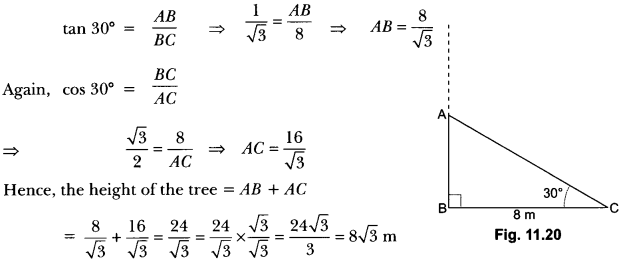Question 3.
The angles of elevation of the top of a tower from two points at a distance of 4 m and 9 m from the base of the tower and in the same straight line with it are complementary. Prove that the height of the tower is 6 m.
Solution: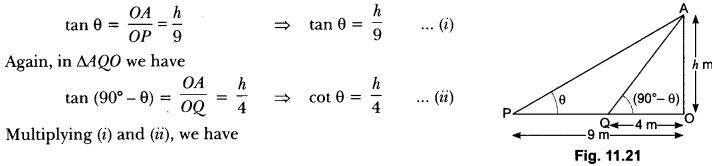Let OA be the tower of height h metre and P, l be the two points at distance of 9 m and 4 m respectively from the base of the tower.
Now, we have OP = 9 m, OQ = 4 m,
Let ∠APO = θ, ∠AQO = (90° – θ)
and OA = h metre (Fig. 11.21)
Now, in ∆POA, we have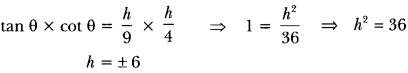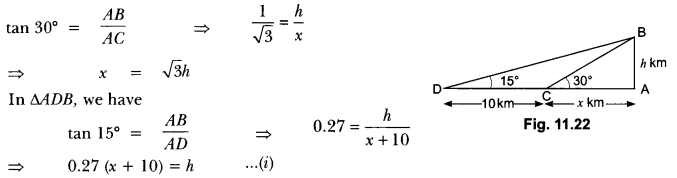Height cannot be negative.
Hence, the height of the tower is 6 metre.

Question 4.
Determine the height of a mountain if the elevation of its top at an unknown distance from the base is 30° and at a distance 10 km further off from the mountain, along the same line, the angle of elevation is 15o. (Use tan 15° = 0.27)
Solution:
Let AB be the mountain of height h kilometres. Let C be a point at a distance of x km, from the base of the mountain such that the angle of elevation of the top at C is 30°. Let D be a point at a distance of 10 km from C such that angle of elevation at D is of 15°.
In MBC (Fig. 11.22), we have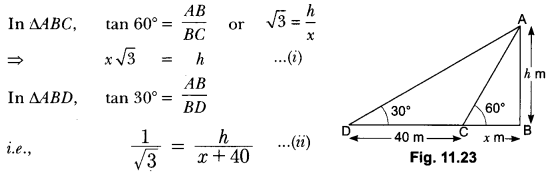Substituting x = √3h in equation (i), we get
⇒ 0.27 ( √3h + 10) = h
= 0.27 × √3h + 0.27 × 10 = h
⇒ 2.7 = h – 0.27 × √3h
⇒ 27 = h (1 – 0.27 × √3)
⇒ 27 = h (1 – 0.46)
⇒ h = $$\frac{2.7}{0.54}$$ = 5
Hence, the height of the mountain is 5 km.

Question 5.
The shadow of a tower standing on a level ground is found to be 40 m longer when the Sun’s altitude is 30° than when it is 60°. Find the height of the tower.
Solution:
In Fig. 11.23, AB is the tower and BC is the length of the shadow when the Sun’s altitude is 60°, i.e., the angle of elevation of the top of the tower from the tip of the shadow is 60° and DB is the length of the shadow, when the angle of elevation is 30°.
Now, let AB be h m and BC be x m.
According to the question, DB is 40 m longer than BC.
So, BD = (40 + x) m
Now, we have two right triangles ABC and ABD.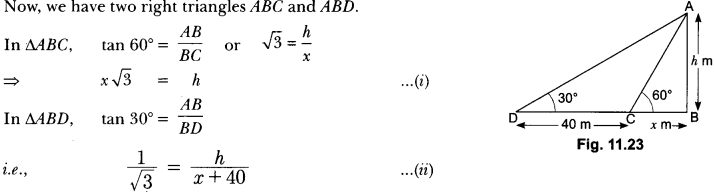Using (i) in (ii), we get (x √3 ) √3 = x + 40, i.e., 3x = x + 40
i.e., x = 20
So, h = 20 √3 [From (i)]
Therefore, the height of the tower is 20 √3 m.

Question 6.
From a point P on the ground, the angle of elevation of the top of a 10m tall building is 30°. A flag is hosted at the top of the building and the angle of elevation of the top of the flagstaff from P is 45o. Find the length of the flagstaff and the distance of the building from the point P. (You may take √3 = 1.732).
Solution: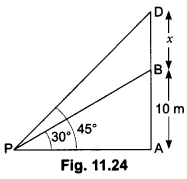In Fig. 11.24, AB denotes the height of the building, BD the flagstaff and P the given point. Note that there are two right triangles PAB and PAD. We are required to find the length of the flagstaff, i.e., BD and the distance of the building from the point P, i.e., PA.
Since, we know the height of the building AB, we will first consider the right ∆PAB.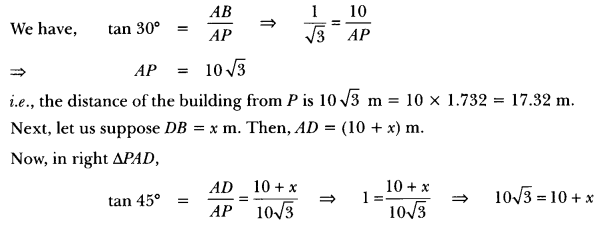i.e., x = 100(√3 – 1) = 7.32
So, the length of the flagstaff is 7.32 m.

Question 7.
A contractor plans to install two slides for the children to play in a park. For the children below the age of 5 years, she prefers to have a slide whose top is at a height of 1.5 m, and is inclined at an angle of 30° to the ground, whereas for elder children, she wants to have a steep slide at a height of 3 m, and inclined at an angle of 60° to the ground. What should be the length of the slide in each case?
Solution:
Let AC be a steep slide for elder children and DE be a slide for younger children. Then AB = 3 m and DB = 1.5 m (Fig. 11.25).
Now, in right angle ∆DBE, we have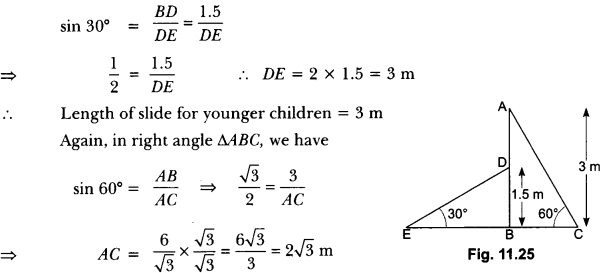So, the length of slide for elder children is 2 √3 m.

Question 8.
A kite is flying at a height of 60 m above the ground. The string attached to the kite is temporarily tied to a point on the ground. The inclination of the string with the ground is 60°. Find the length of the string, assuming that there is no slack in the string.
Solution:
Let AB be the horizontal ground and K be the position of the kite and its height from the ground is 60 m and let length of string AK be x m. (Fig. 11.26)
∠KAB = 60°
Now, in right angle ∆ABK we have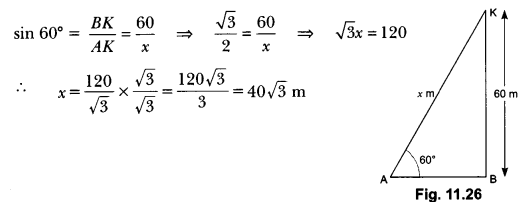So, the length of string is 40 √3 m.

Question 9.
A 1.5 m tall boy is standing at some distance from a 30 m tall building. The angle of elevation from his eyes to the top of the building increases from 30° to 60° as he walks towards the building. Find the distance he walked towards the building.
Solution:
Let AB be the building and PQ be the initial position of the boy (Fig. 11.27) such that
∠APR = 30°
and AB = 30 m
Now, let the new position of the boy be P’Q’ at a distance QQ’.
Here, ∠AP’R = 60°
Now, in ∆ARP, we have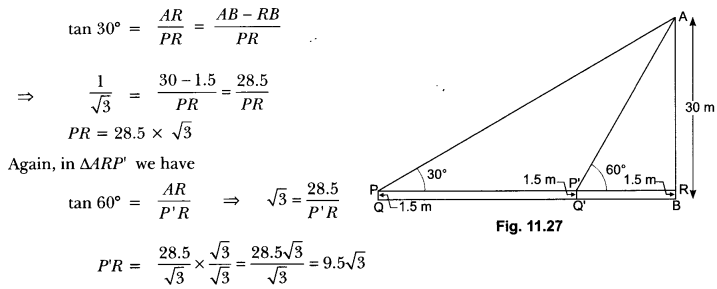Therefore, required distance, QQ = PP’ = PR – P’R
= 28.5 √3 – 9.5 √3 = 19√3
Hence, distance walked by the boy is 19√3 m.

Question 10.
From a point on a bridge across a river, the angles of depression of the banks on opposite sides of the river are 30° and 45° respectively. If the bridge is at a height of 3 m from the banks, find
the width of the river.
Solution:
In Fig. 11.28, A and B represent points on the bank on opposite sides of the river, so that AB is the width of the river. P is a point on the bridge at a height of 3 m, i.e., DP = 3m. We are interested to determine the width of the river, which is the length of the side AB of the ∆APB.
In right ∆ADP, ∠A = 30°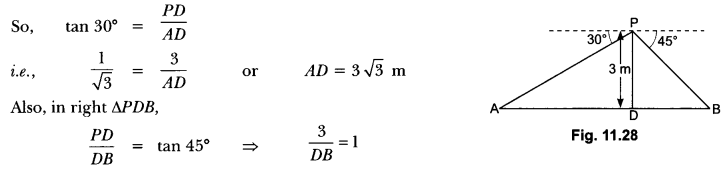∴ DB = 3m
Now, AB = BD + AD = 3 + 3 √3 = 3 (1 + √3) m
Therefore, the width of the river is 3(√3 + 1) m.

Question 11.
As observed from the top of a 75 m high lighthouse from the sea-level, the angles of depression of two ships are 30° and 45°. If one ship is exactly behind the other on the same side of the lighthouse, find the distance between the two ships.
Solution: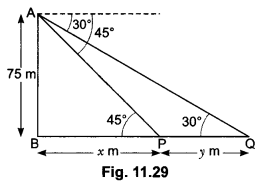Let AB be the lighthouse of height 75 m and P, Q be the position of the two ships whose angles of depression are 45° and 30°, respectively (Fig. 11.29). Let BP = x m and PQ = y m, we have
∠APB = 45° and ∠AQB = 30°
Now, in ∆ABP we have
tan 45° = $$\frac{AB}{BP}$$
⇒ 1 = $$\frac{70}{x}$$
⇒ x = 75 m …..(i)
Again, in ∆ABQ we have
tan 30° = $$\frac{AB}{BQ}$$
⇒ $$\frac{1}{\sqrt{3}}$$ = $$\frac{75}{x+y}$$
⇒ x + y = 75 √3 ……(ii)
From (i) and (ii), we have
75 + y = 75 √3
y = 75 √3 – 75
⇒ y = 75(√3 – 1)
Hence, the distance between two ships is 75(√3 – 1) metres.

Question 12.
Two ships are there in the sea on either side of a light house in such a way that the ships and the light house are in the same straight line. The angles of depression of two ships as observed from the top of the light house are 60° and 45°. If the height of the light house is 200 m, find the distance between the two ships. (Use √3 = 1.73]
Solution:
Let the distance between the two ships be d.
Let the distance of one ship from the light house be x metres. Then, the distance of the other ship from the light house will be (d – x) metres.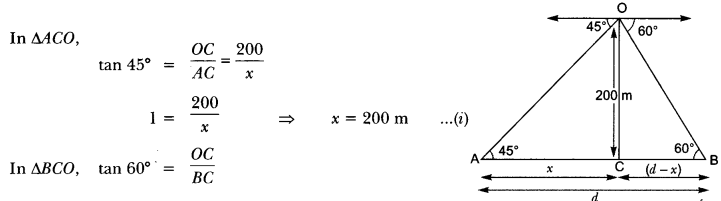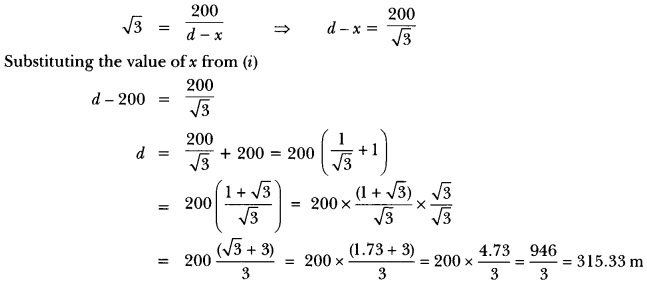Question 13.
The angle of elevation of an aeroplane from a point on the ground is 60°. After a flight of 30 seconds the angle of elevation becomes 30°. If the aeroplane is flying at a constant height of 3000 √3 m, find the speed of the aeroplane.
Solution:
Let A be point of observation and P and Q be positions of the plane. Let ABC be the line through A and it is given that angles of elevation from point A to two positions P and Q are 60° and 30°.
∠PAB = 60°, ∠QAB = 30°
Height = 3000 √3 m
So, in ∆ABP, we have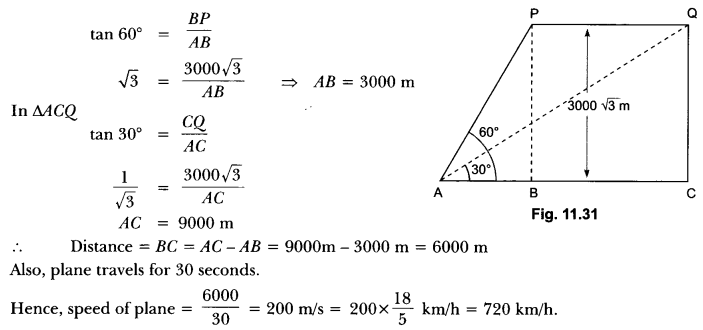Question 14.
From the top of a 60 m high building, the angles of depression of the top and the bottom of a tower are 45° and 60° respectively. Find the height of the tower. [Take √3 = 1.73]
Solution:
Let the height of the building be AE = 60 m, the height of the tower is ‘h’. The distance between the base of the building and the tower be ‘d’.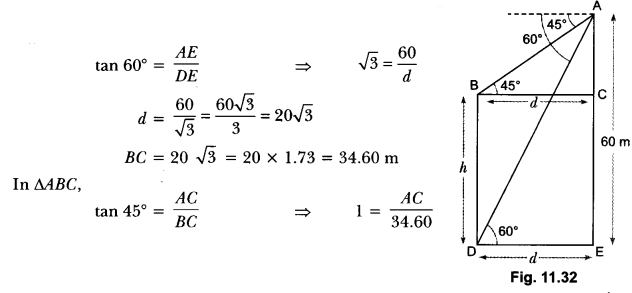⇒ AC = 34.60 m
Now, height of tower = AE – AC = 60 – 34.60 = 25.4 m

Question 15.
Two ships are approaching a light-house from opposite directions. The angles of depression of the two ships from the top of the light-house are 30° and 45°. If the distance between the two ships is 100 m, find the height of the light-house. (Use √3 = 1.732]
Solution:
Let AD be the light house and its height be h. The distance of one ship from the light house is x and that of other ship is 100 – x.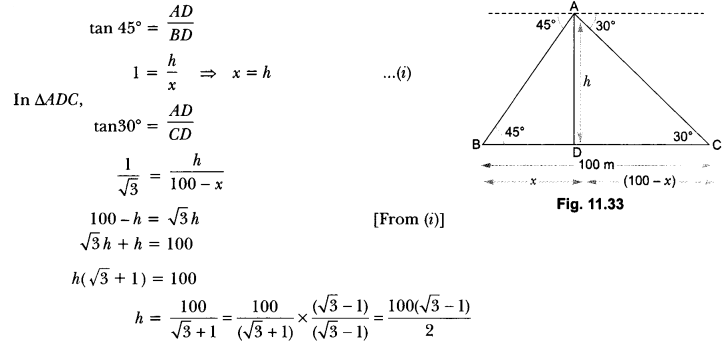= 50 × (1.732 – 1)
h = 36.6 m

Question 16.
Two men on either side of a 75 m high building and in line with base of building observe the angle of elevation of the top of the building as 30° and 60°. Find the distance between the two men. (Use √3 = 1.73)
Solution: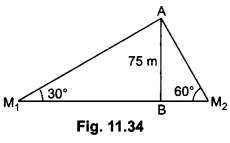(i) In ∆ABM1
$$\frac{A B}{B M_{1}}$$ = tan 30° = $$\frac{1}{\sqrt{3}}$$
⇒ BM1 = 75 √3 m

(ii) In ∆ABM2
$$\frac{A B}{B M_{2}}$$ = tan 60° = √3
⇒ BM2 = $$\frac{75}{\sqrt{3}}$$ = 25 √3 m
∴ M1 M2 = M1B + BM2
= 75√3 + 25 √3 = 100 √3 m = 173 m
∴ Distance between two men = 173 m.

Question 17.
A moving boat is observed from the top of a 150 m high cliff moving away from the cliff. The angle of depression of the boat changes from 60° to 45° in 2 minutes. Find the speed of the boat in m/h.
Solution: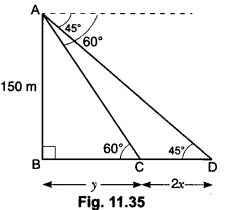Let the speed of boat be x m/min
∴ CD = 2x
In ∆ABC
$$\frac{150}{y}$$ = tan 60°
⇒ y =$$\frac{150}{\sqrt{3}}$$ = 50√3 m
In ∆ABD
$$\frac{150}{y+2 x}$$ = tan 45°
⇒ 150 = 50√3 + 2x
⇒ x = 25(3 – √3)
Speed = 25(3 – √3 ) m/min
∴ = 25 × 60 (3 – √3) m/h = 1500 (3 – √3) m/h

### Some Applications of Trigonometry Class 10 Extra Questions Long Answer Type

Question 1.
From a point on the ground, the angles of elevation of the bottom and the top of a transmission tower fixed at the top of a 20 m high building are 45° and 60°, respectively. Find the height of the tower.
Solution:
Let AB be a building of height 20 m and BC be the transmission tower of height x m and D be any point on the ground (Fig. 11.36).
Here, ∠BDA = 45° and ∠ADC = 60°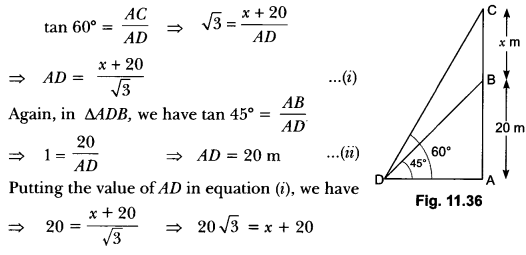⇒ x = 20√3 – 20 = 20 (√3 – 1) = 20 (1.732 – 1) = 20 × 0.732 = 14.64 m
Hence, the height of tower is 14.64 m.

Question 2.
A statue, 1.6 m tall, stands on the top of a pedestal. From a point on the ground, the angle of elevation of the top of the statue is 60° and from the same point, the angle of elevation of the bottom of the pedestal is 45°. Find the height of the pedestal.
Solution:
Let AB be the pedestal of height h metres and BC be the statue of height 1.6 m (Fig. 11.37).
Let D be any point on the ground such that,
∠BDA = 45° and ∠CDA = 60°
Now, in ∆BDA, we have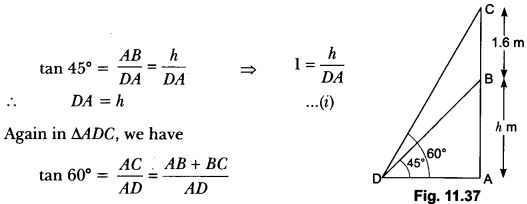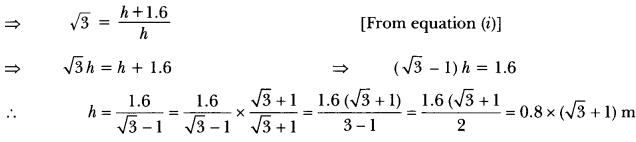Hence, height of the pedestal is 0.8 (√3 + 1) m.

Question 3.
From the top of a 7 m high building, the angle of elevation of the top of a cable tower is 60° and the angle of depression of its foot is 45°. Determine the height of the tower.
OR
From the top of a 7 m high building, the angle of elevation of the top of a tower is 60° and the angle of depression of its foot is 45°. Find the height of the tower. (Use √3 = 1.732]
Solution:Let PQ be the building of height 7 metres and AB be the cable tower. Now it is given that the angle of elevation of the top A of the tower observed from the top P of building is 60° and the angle of depression of the base B of the tower observed from P is 45° (Fig. 11.38).
So, ∠APR = 60° and ∠QBP = 45°
Let QB = x m, AR = h m then, PR = x m
Now, in ∆APR, we have
tan 60° = $$\frac{AR}{PR}$$
⇒ √3 = $$\frac{h}{x}$$
⇒ √3x = h
⇒ h = √3x ……(i)
Again, in ∆PBQ we have
tan 45o = $$\frac{PQ}{QB}$$
⇒ 1 = $$\frac{7}{x}$$
⇒ x = 7 ……(ii)
Putting the value of x in equation (i), we have
h = √3 × 7 = 7 √3
i.e., AR = 7 √3 metres
So, the height of tower = AB = AR + RB = 7 √3 + 7 = 7(√3 + 1) m.

Question 4.
At a point, the angle of elevation of a tower is such that its tangent is $$\frac{5}{12}$$ On walking 240 m to the tower, the tangent of the angle of elevation becomes $$\frac{3}{4}$$. Find the height of the tower.
Solution:
In the Fig. 11.39, let AB be the tower, C and D be the positions of observation from where given that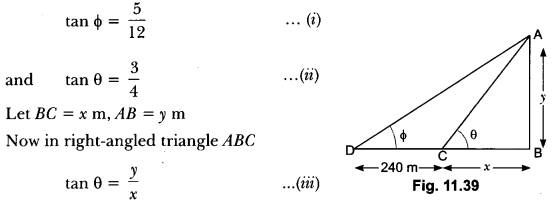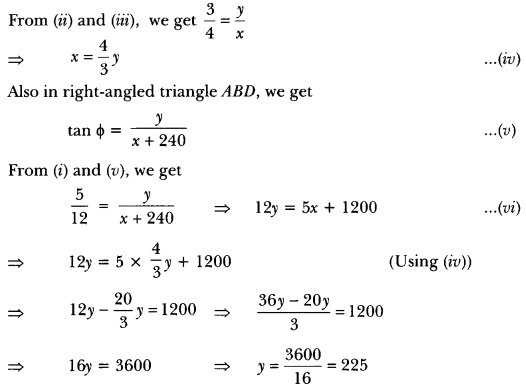Hence, the height of the tower is 225 metres.

Question 5.
A 1.2 m tall girl spots a balloon moving with the wind in a horizontal line at a height of 88.2 m from the ground. The angle of elevation of the balloon from the eyes of the girl at any instant is 60°. After some time, the angle of elevation reduces to 30° (Fig. 11.40). Find the distance travelled by the balloon during the interval.
Solution:
Let A and B be two positions of the balloon and G be the point of observation. (eyes of the girl)
Now, we have
AC = BD = BQ – DQ = 88.2 m – 1.2 m = 87 m .
∠AGC = 60°, ∠BGD = 30°
Now, in ∆AGC, we have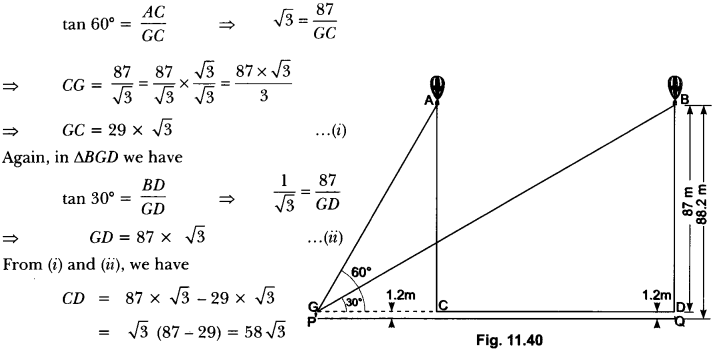Hence, the balloon travels 58 √3 metres.

Question 6.
A straight highway leads to the foot of a tower. A man standing at the top of the tower observes a car at an angle of depression of 30°, which is approaching the foot of the tower with a uniform speed. Six seconds later, the angle of depression of the car is found to be 60°. Find the time taken by the car to reach the foot of the tower from this point.
Solution:
Let OA be the tower of height h, and P be the initial position of the car when the angle of depression is 30°.
After 6 seconds, the car reaches to such that the angle of depression at Q is 60°. Let the speed of the car be v metre per second. Then,
PQ = 6υ (∵ Distance = speed × time)
and let the car take t seconds to reach the tower OA from Q (Fig. 11.41). Then, OQ = υt metres.
Now, in ∆AQO, we have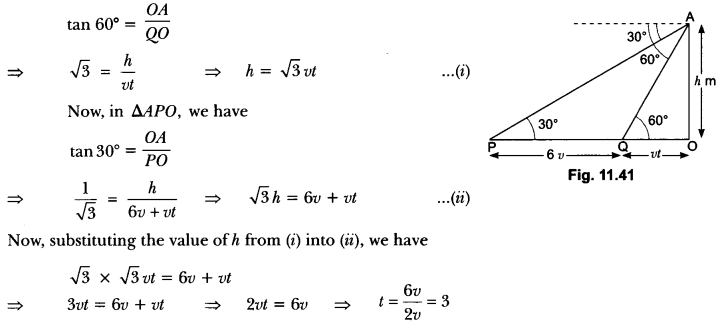Hence, the car will reach the tower from Q in 3 seconds.

Question 7.
In Fig. 11.42, ABDC is a trapezium in which AB || CD. Line segments RN and LM are drawn parallel to AB such that AJ = JK = KP. If AB = 0.5 m and AP = BQ = 1.8 m, find the lengths of AC, BD, RN and LM.
Solution:
We have,
AP = 1.8 m
AJ = JK = KP = 0.6 m
AK = 2AJ = 1.2 m
In ∆ARJ and ∆BNJ’ we have
AJ = BJ, ∠ARJ = ∠BNJ = 60°
and ∠AJR = ∠BJ’N = 90°
∴ ∆ARJ ≅ ∆BNJ
⇒ RJ = NJ (By AAS congruence criterion)
Similarly, ∆ALK ≅ ∆BMK”
⇒ LK = MK”
In ∆ARJ,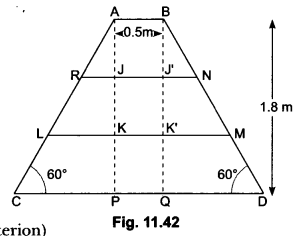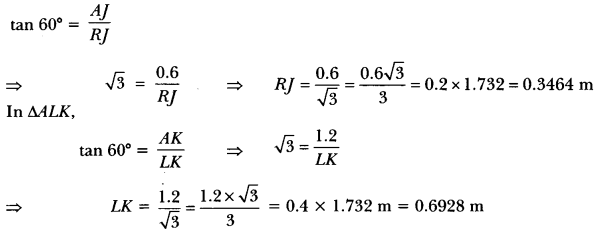Since ∆ACP ≅ ∆BDQ
So, BD = AC = 2.0784 m
Now, RN = RJ + JJ + J’N
= 2RJ + AB [∵ RJ = J’ N and JJ = AB]
= 2 × 0.3464 +0.5 = 1.1928 m
Length of step LM = LK + KK + KM
= 2LK + AB [∵ LK = K M and KK = AB]
= 2 × 0.6928 + 0.5 = 1.8856 m
Thus, length of each leg = 2.0784 m = 2.1 m
Length of step RN = 1.1928 m = 1.2 m
and, length of step LM = 1.8856 m = 1.9 m

Question 8.
Two poles of equal heights are standing opposite to each other on either side of the road, which is 80 m wide. From a point between them on the road, the angles of elevation of the top of the poles are 60° and 30°, respectively. Find the height of the poles and the distances of the point from the poles.
Solution: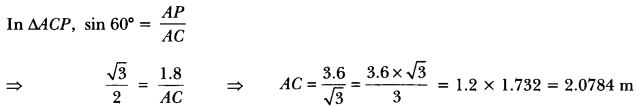Let AB and CD be two poles of equal height h metre and let P be any point between the poles, such that
∠APB = 60° and ∠DPC = 30°
The distance between two poles is 80m.(Given)
Let AP = x m, then PC = (80 – x) m.
h’m Now, in ∆APB, we have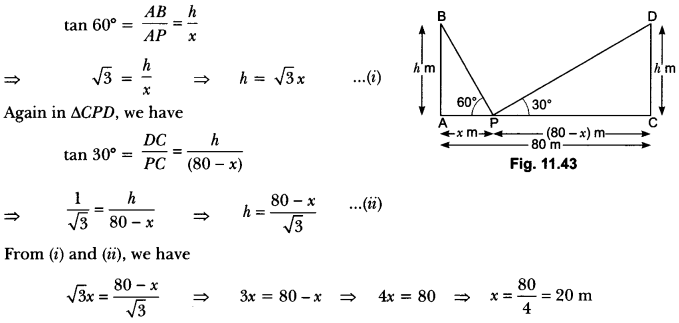Now, putting the value of x in equation (i), we have
h = √3 × 20 = 20 √3
Hence, the height of the pole is 20 √3 m and the distance of the point from first pole is 20 m and that of the second pole is 60 m.

Question 9.
A TV tower stands vertically on a bank of a canal. From a point on the other bank directly opposite the tower, the angle of elevation of the top of the tower is 60°. From another point 20 m away from this point on the line joining this point to the foot of the tower, the angle of elevation of the top of the tower is 30° (Fig. 11.44). Find the height of the tower and the width of the canal.
Solution:
Let height of the tower be h metres and width of the canal be x metres, so AB = h m and BC = x m
Now in ∆ABC, we have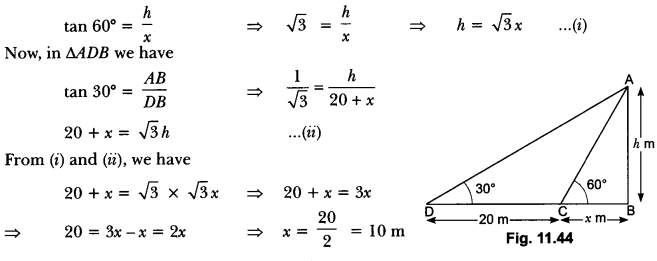Now, putting the value of x in equation (i), we have
h = √3 × 10 = 10√3
⇒ h = 10 73 m
Hence, height of the tower is 10 √3 m and width of the canal is 10 m.

Question 10.
A person standing on the bank of a river observes that the angle of elevation of the top of a tree standing on the opposite bank is 60°. When he moves 40 metres away from the bank, he finds the angle of elevation to be 30°. Find the height of the tree and the width of the river.
Solution:
Let AB be the tree of height metres standing on the bank of a river. Let C be the position of man standing on the opposite bank of the river such that BC = x m. Let D be the new position of the man. It is given that CD = 40 m and the angles of elevation of the top of the tree at C and D are 60° and 30°, respectively, i.e.,
∠ACB = 60° and ∠ADB = 30°.
In ∆ACB, we have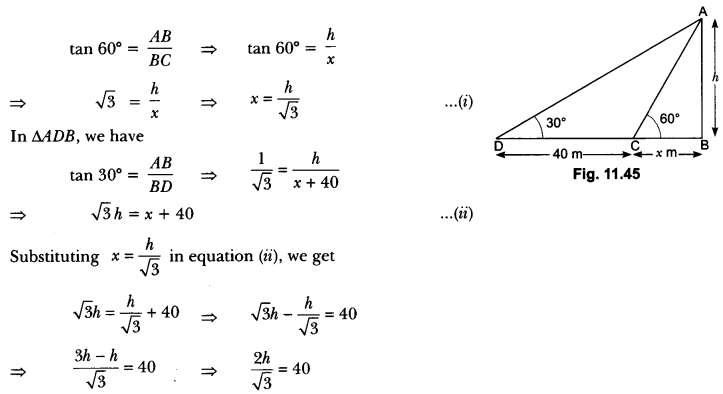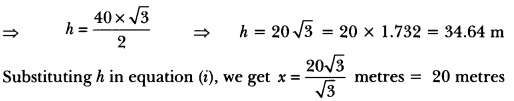Hence, the height of the tree is 34.64 m and width of the river is 20 m.

Question 11.
The angles of elevation and depression of the top and bottom of a lighthouse from the top of a building, 60 m high, are 30° and 60° respectively. Find
(i) the difference between the heights of the lighthouse and the building.
(ii) distance between the lighthouse and the building.
Solution:
Let AB be the building and CE be the lighthouse (Fig. 11.46).
In right-angled ∆ABC,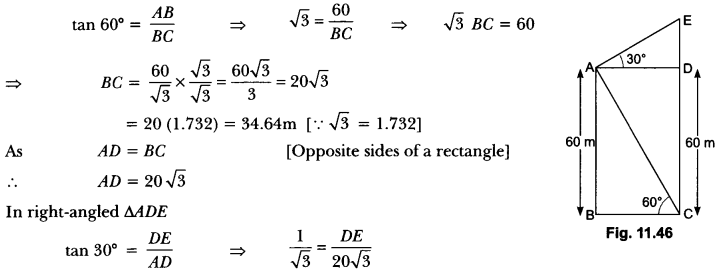= √3 DE = 20 √3
⇒ DE = 20
∴ (i) Difference between the heights of lighthouse and building = EC – DC = DE = 20 m
and (ii) Distance between the lighthouse and the building = BC = 34.64 m.

Question 12.
In Fig. 11.47, from the top of a building AB, 60 metres high, the angles of depression of the top and bottom of a vertical lamp post CD are observed to be 30° and 60°, respectively. Find
(i) the horizontal distance between AB and CD.
(ii) the height of the lamp post.
Solution:
Given AB is the building and CD is the vertical lamp post. Then, DE is the horizontal distance between AB and CD. Let CD = h metres.
Then
∠EDB = 30° and ∠ACB = 60°, AE = CD = h metres and EB = (60 -h) m
Now, in ∆ABC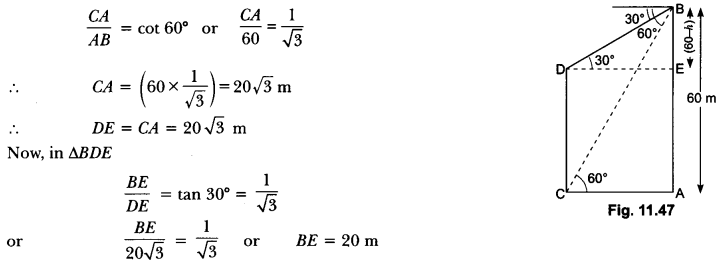⇒ 60 – h = 20, i.e., h = 40 m
∴ (i) Horizontal distance between AB and CD = 20 √3 m = 20 × 1.732 = 34.64 m
(ii) The height of lamp post = 40 m

Question 13.
A boy standing on a horizontal plane finds a bird flying at a distance an elevation of 30°. A girl standing on the roof of 20 metre high building, finds the angle of elevation of the same bird to be 45°. Both the boy and the girl are on opposite sides of the bird. Find the distance of bird from the girl.
Solution:
Let B be the position of bird. O and P be the positions of boy and girl respectively and PQ be the building
We have, ∠AOB = 30° and ∠BPM = 45°
Now, in ∆AOB we have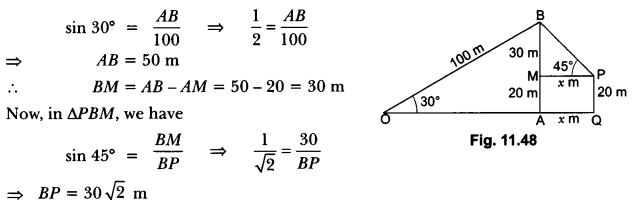Hence, distance of bird from girl is 30 √2 m.

Question 14.
The angles of depression of the top and the bottom of a 8 m tall building from the top of a multi-storeyed building are 30° and 45°, respectively. Find the height of the multi-storeyed building and the distance between the two buildings.
Solution:
In Fig. 11.49, PC denotes the multi-storeyed building and AB denotes the 8 m tall building. We are interested to determine the height of the multi-storeyed building, i.e., PC and the distance between the two buildings, i.e., AC.
Let PD = x m
In right ∆PDB, we have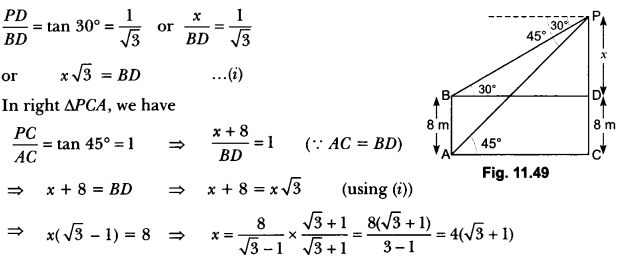From (i), BD = [4(√3 + 1)] √3 = 4(3 + √3 )]
So, the height of the multi-storeyed building is {4( √3 + 1) +8} m = 4(3 + √3) m
and the distance between the two buildings is also 4(3 + √3) m.

Question 15.
The angle of elevation of the top of a tower at a distance of 120 m from a point A on the ground is 45°. If the angle of elevation of the top of a flagstaff fixed at the top of the tower, at A is 60°, then find the height of the flagstaff. [Use √3 = 1.73]
Solution: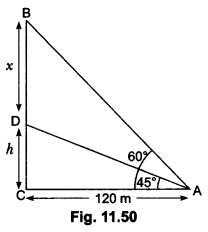Let CD be the tower which is at a distance of 120m from A.
BD = x be the length of flagstaff.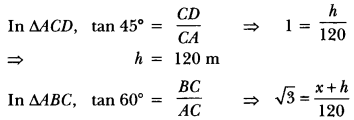120 √3 = x + 120
= 120 × 1.73 – 120
x = 120 (1.73 – 1) = 120 × 0.73
x = 87.6 m

Question 16.
The angle of elevation of the top of a building from the foot of the tower is 30° and the angle of elevation of the top of the tower from the foot of the building is 60°. If the tower is 50 m high, find the height of the building.
Solution:
Let AB be the building of height h m and CD be the tower of height 50 m. We have,
∠ACB = 30° and ∠DAC = 60°
Now, in ∆ACD, we have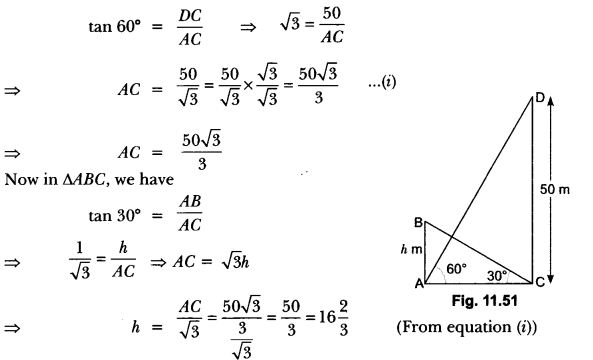Hence, the height of the building is 16$$\frac{2}{3}$$m.

Question 17.
A vertical tower stands on a horizontal plane and is surmounted by a flagstaff of height 5 m. From a point on the ground the angles of elevation of the top and bottom of the flagstaff are 60° and 30° respectively. Find the height of the tower and the distance of the point from the tower. (Take √3 = 1.732)
Solution:
Let height of tower be x m and distance of
point from tower be y m.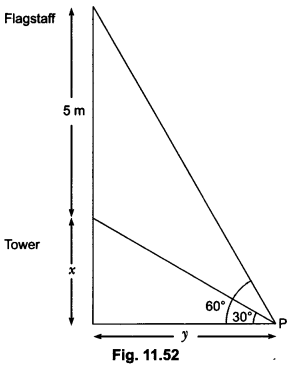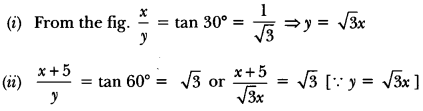= x + 5 = 3x
⇒ x = $$\frac{5}{2}$$ = 2.5
Height of tower = 2.5 m
Distance of point from tower = y = √3x
= (2.5 x 1.732) or 4.33 m

Question 18.
From the top of a tower, 100 m high, a man observes two cars on the opposite sides of the tower and in same straight line with its base, with angles of depression 30° and 45o. Find the distance between the cars.[Take √3 = 1.732]
Solution:
Let AQ be the tower of height 100m. Car B and Car Care in opposite direction and at distance
of x m and y m respectively.
In ∆ABO,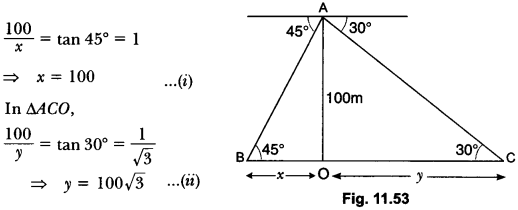Distance between the cars = x + y
= 100 + 100√3 [From equation (i) and (ii)]
= 100 (1 + √3 )
= 100 (1 + 1.732) = 273.2 m

### Some Applications of Trigonometry Class 10 Extra Questions HOTS

Question 1.
A man standing on the deck of a ship, which is 10 m above the water level, observes the angle of elevation of the top of a hill as 60° and the angle of depression of the base of the hill as 30°. Calculate the distance of the hill from the ship and the height of the hill.
Solution:
In Fig. 11.54, let C represents the position of the man on the deck of the ship, A represents the top of hill and D its base.
Now in right-angled triangle CWD,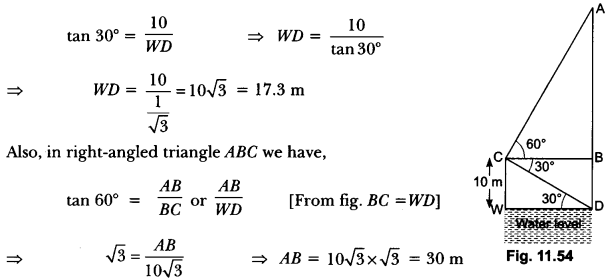Now, AD = AB + BD = 30 m + 10 m = 40 m.
Therefore, the distance of the hill from the ship = 17.3 m and the height of the hill = 40 m

Question 2.
A spherical balloon of radius r subtends an angle θ at the eye of an observer. If the angle of elevation of its centre is Ø, find the height of the centre of the balloon.
Solution:
In Fig. 11.55, O is the centre of balloon, whose radius OP = rand ∠PAQ = 0. Also, ∠OAB = Ø.
Let the height of the centre of the balloon be h. Thus OB = h.
Now, from ∆OAP,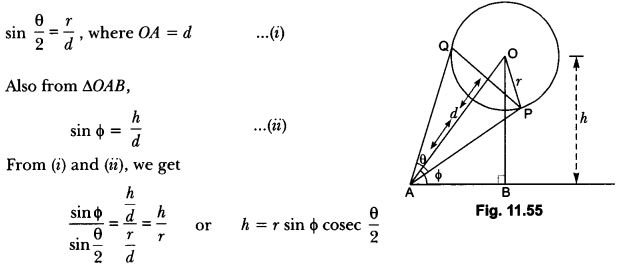Question 3.
From a window (h metres high above the ground) of a house in a street, the angles of elevation and depression of the top and the foot of another house on the opposite side of the street are θ and Ø respectively. Show that the height of the opposite house is h (1 + tan θ cot Ø).
Solution:
Let W be the window and AB be the house on the opposite side.
Then, WP is the width of the street (Fig. 11.56).
Let AP = h’ m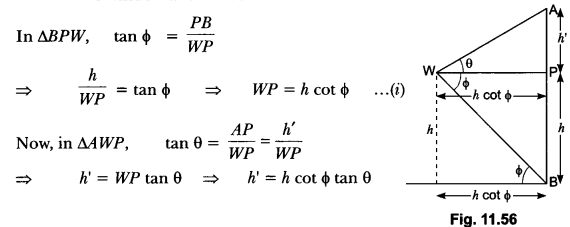⇒ h’ = WP tan θ
⇒ h’ = h cot Ø tan θ
∴ Height of house = h’ + h
= h cot Ø tan θ + h = h (1 + tan θ cot Ø)

Question 4.
The angle of elevation of a jet plane from a point A on the ground is 60°. After a flight of 15 seconds, the angle of elevation changes to 30°. If the jet plane is flying at a constant height of
1500√3 m find the speed of the jet plane.
Solution:
Let P and Q be the two positions of the plane and let A be the point of observation. Let ABC be the horizontal line through A. It is given that angles of elevation of the plane in two positions P and Q from a point A are 60° and 30°, respectively,
Then, ∠PAB = 60°, ∠QAB = 30°
It is also given that PB = 1500√3 metres
In ∆ABP, we have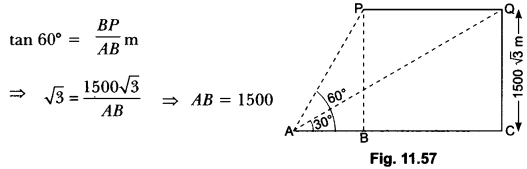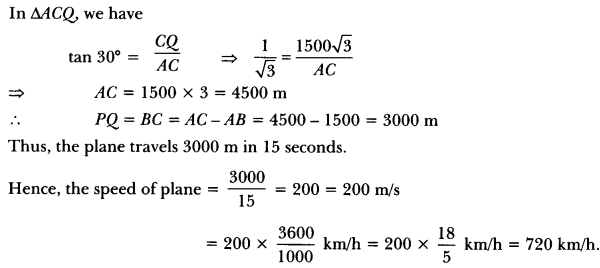Question 5.
If the angle of elevation of a cloud from a point h metres above a lake is a and the angle of depression of its reflection in the lake is B, prove that the height of the cloud is $$\frac { h(tanß-tanα) }{ tan ß – tan α }$$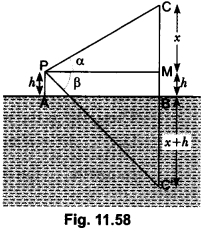Solution:
Let AB be the surface of the lake and let P be a point of observation (Fig. 11.58) such that AP = h metres. Let C be the position of the cloud and C’ be its reflection in the lake. Then, CB = C’ B. Let PM be perpendicular from P on CB. Then, ∠CPM = a and ∠MPC’ = B.
Let CM = x.
Then, CB = CM + MB = CM + PA = x + h
In ∆CPM, we have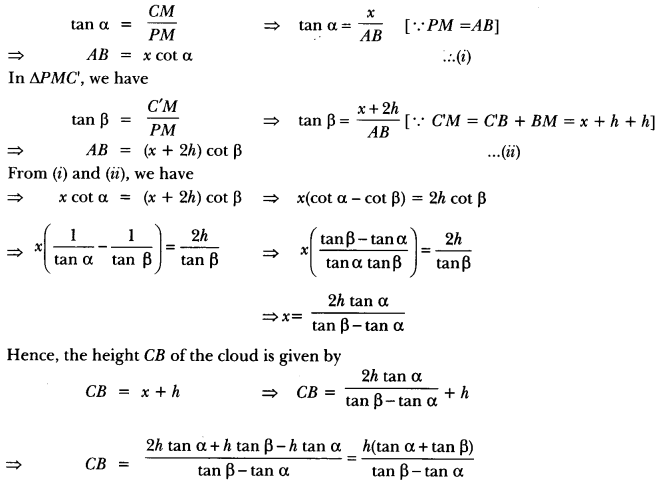Question 6.
The shadow of a tower at a time is three times as long as its shadow when the angle of elevation of the sun is 60°. Find the angle of elevation of the sun at the time of the longer shadow.
Solution:
Let AB be the flagstaff and BC be the length of its shadow when the Sun rays meet the ground at an angle of 60°. Let o be the angle between the Sun rays and the ground when the length of the shadow of the flagstaff is BD. Let h be the height of the flagstaff (Fig. 11.59).
Let BC = x
∴ BD = 3x and CD = 2x
In ∆ABC, we have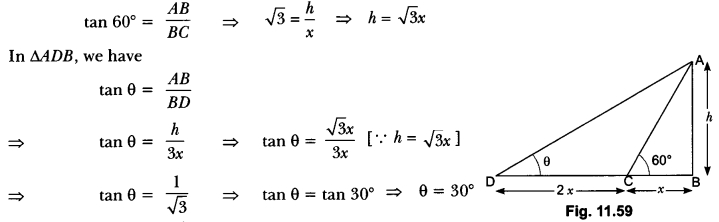Question 7.
From an aeroplane vertically above a straight horizontal plane, the angles of depression of two consecutive kilometre stones on the opposite sides of the aeroplane are found to be α and β. Show that the height of the aeroplane is = $$\frac { tanα.tanβ }{ tanα+tanβ }$$
Solution:
In Fig. 11.60, let P be the position of plane, A and B be the positions of two stones one kilometre apart. Angles of depression of stones A and B are a and ß respectively.
Let PC = h.
In right-angled triangle ACP, we haveQuestion 8.
The angle of elevation of a cliff from a fixed point is θ. After going up a distance of k metres towards the top of the cliff at an angle of Ø, it is found that the angle of elevation is α. Show that the height of the cliff is $$\frac {k(cos\phi-sin\phi cot\alpha)}{cot\theta-cot\alpha}$$ metres.
Solution:
Let AB be the cliff and O be the fixed point such that ∠AOB = θ. Let ∠AOC = Ø and OC = k m.
From C, draw CD and CE perpendiculars on AB and OA, respectively. Now, ∠DCB = a. Let h be the height of the cliff AB.
In ∆COE, we have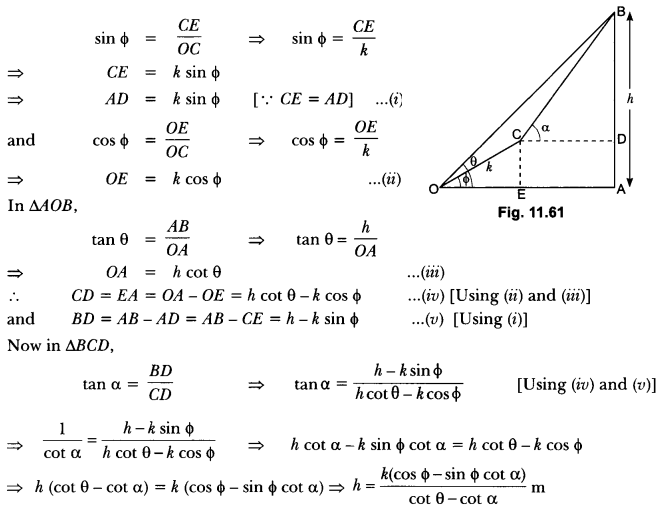Hence Proved.

Question 9.
The angle of elevation of the top of a tower from a point A due south of the tower is a and from B due east of the tower is β. if AB = d, show that the height of the tower is $$\frac {d}{\sqrt{{cot}^{2}}\alpha+{cot}^{2}\beta}$$.
Solution:
Let OC be the tower. Let height of tower OC be h. A and B be two points due south and east of tower at O.
In ∠AOC, ∠O = 90°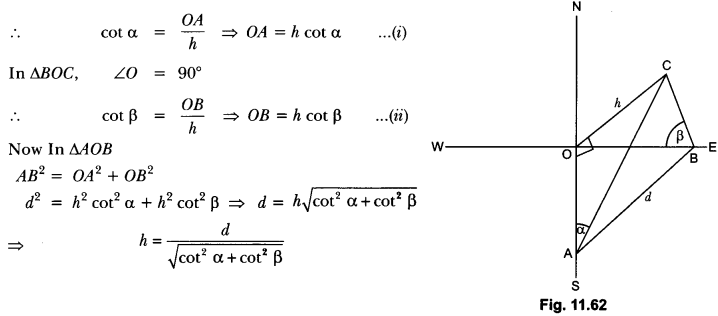Question 10.
From the top of a hill, the angles of depression of two consecutive kilometre stones due east are found to be 45° and 30° respectively. Find the height of the hill.
Solution:
Let the height of the hill be hm, C and D are two consecutive stones having distance 1000 m between them and AC = x m.
In ∆ABC,
tan 45° = $$\frac{h}{x}$$
⇒ x = h ….(i)
In ∆ABD,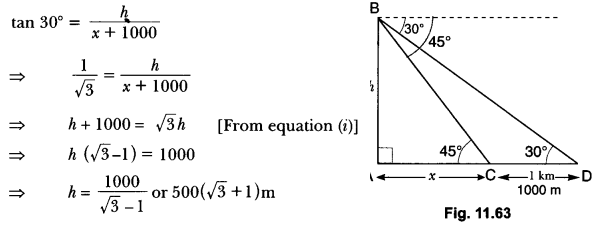Hence, the height of the hill = 500(√3 + 1)m.